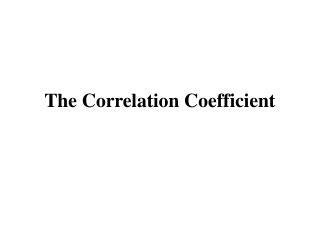DownloadDownload PresentationThe Correlation Coefficient

# The Correlation Coefficient

Télécharger la présentation## The Correlation Coefficient

- - - - - - - - - - - - - - - - - - - - - - - - - - - E N D - - - - - - - - - - - - - - - - - - - - - - - - - - -
##### Presentation Transcript

1. The Correlation Coefficient

2. Social Security Numbers

3. A Scatter Diagram

4. The Point of Averages • Where is the center of the cloud? • Take the average of the x-values and the average of the y-values; this is the point of averages. • It locates the center of the cloud. • Similarly, take the SD of the x-values and the SD of the y-values.

5. Examples

6. The Correlation Coefficient • An association can be stronger or weaker. • Remember: a strong association means that knowing one variable helps to predict the other variable to a large extend. • The correlation coefficient is a numerical value expressing the strength of the association.

7. The Correlation Coefficient • We denote the correlation coefficient by r. • If r = 0, the cloud is completely formless; there is no correlation between the variables. • If r = 1, all the points lie exactly on a line (not necessarily x = y) and there is perfect correlation.

8. Strong and Weak

9. The Correlation Coefficient • What about negative values? • The correlation coefficient is between –1 and 1, negative shows negative association, positive indicates positive association. • Note that –0.90 shows the same degree of association as +0.90, only negative instead of positive.

10. Computing the Correlation Coefficient • Convert each variable to standard units. • The average of the products gives the correlation coefficient r. r = average of (x in standard units)  (y in standard units)

11. Example We mustfirstconvert to standard units. Find the average and the SD of the x-values: average = 4, SD = 2. Find the deviation: subtract the average from each value, and divide by the SD. Then do the same for the y-values.

12. Example

13. Example • Finally, take the average of the products • In this example, r = 0.40. r = average of (x in standard units)  (y in standard units)

14. The SD line • If there is some association, the points in the scatter diagram cluster around a line. But around which line? • Generally, this is the SDline. It is the line through the point of averages. • It climbs at the rate of one vertical SD for each horizontal SD. • Its slope is (SD of y) / (SD of x) in case of a positive correlation, and –(SD of y) / (SD of x) in case of a negative correlation.

15. Five-point Summary • Remember the five-point summary of a data set: minimum, lower quartile, median, upper quartile, and maximum. • A five-point summary for a scatter plot is: average x-values, SD x-values, average y-values, SD y-values, and correlation coefficient r.